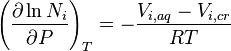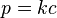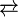# Solubility

The solubility of a chemical substance is a physical property referring to the ability of that substance, called the solute, to dissolve in a solvent. It has been called one of the "characteristic properties" of a substance, meaning that it is commonly used to describe the substance, to indicate its polarity, to distinguish it from other substances, and to serve as a guide to applications of that substance.

Solubility is measured in terms of the maximum amount of solute that can be dissolved in a solvent at chemical equilibrium. The resultant solution is called a saturated solution. The solubilities of substances range widely, from those that are infinitely soluble, such as ethanol in water, to those that are poorly soluble, such as silver chloride in water.

Knowledge of the solubilities of substances is valuable for both research and practical applications. For example, it is useful for the separation of mixtures, the processing of ores, the formulation of pharmaceuticals, and the transport of pollutants.

## Terminology

In a solution, the solvent is generally a liquid, which can be a pure substance or a mixture. The species that dissolves (that is, the solute) can be a gas, liquid, or solid. The term insoluble is often applied to poorly soluble compounds.

Certain liquids are soluble in all proportions with a given solvent, such as ethanol in water. This property is known as miscibility. Under certain conditions, the equilibrium solubility can be exceeded to give a so-called supersaturated solution, which is metastable.

## Molecular view

Solubility occurs under dynamic equilibrium, which means that solubility results from the simultaneous and opposing processes of dissolution and precipitation. The solubility equilibrium occurs when the two processes proceed at the same rate.

The solubility equilibrium is relatively straightforward for covalent substances such as benzene. When dissolved in water, the benzene molecules remain intact but interact with and are generally surrounded by molecules of water. When, however, an ionic compound such as sodium chloride (NaCl) dissolves in water, the sodium chloride lattice dissociates into individual ions that are solvated or surrounded by water molecules. Nonetheless, NaCl is said to dissolve in water, because evaporation of the solvent returns crystalline NaCl.

The term "dissolving" is sometimes applied to an irreversible chemical reaction, as with iron in nitric acid, but in such a case the thermodynamic concept of solubility does not apply.

When a solute dissolves, it may form several species in the solution. For example, an aqueous suspension of ferrous hydroxide, Fe(OH)2, will contain the series [Fe(H2O)6−x(OH)x](2−x)+ as well as other oligomeric species. Furthermore, the solubility of ferrous hydroxide and the composition of its soluble components depends on pH. In general, solubility in the solvent phase can be given only for a specific solute that is thermodynamically stable, and the value of the solubility will include all the species in the solution. (In the example above, it would include all the iron-containing complexes.)

## Factors affecting solubility

Solubility is defined for specific forms of each substance. For example, the solubilities of aragonite and calcite in water are expected to differ, even though they are both polymorphs of calcium carbonate and have the same chemical formula.

The solubility of one substance dissolving in another is determined by the balance of intermolecular forces between the solvent and solute, and the entropy change that accompanies the solvation. Factors such as temperature and pressure will alter this balance, thus changing the solubility.

Solubility may also strongly depend on the presence of other species dissolved in the solvent, for example, complex-forming anions (ligands) in liquids. Solubility will also depend on the excess or deficiency of a common ion in the solution, a phenomenon known as the common-ion effect. To a lesser extent, solubility will depend on the ionic strength of liquid solutions. The last two effects can be quantified using the equation for solubility equilibrium.

For a solid that dissolves in a redox reaction, solubility is expected to depend on the potential (within the range of potentials under which the solid remains the thermodynamically stable phase). For example, solubility of gold in high-temperature water is observed to be almost an order of magnitude higher when the redox potential is controlled using a highly oxidizing Fe3O4-Fe2O3 redox buffer than with a moderately oxidizing Ni-NiO buffer.

Solubility (metastable) also depends on the physical size of the crystal or droplet of solute (or, strictly speaking, on the specific or molar surface area of the solute). For highly defective crystals, solubility may increase with the increasing degree of disorder. Both of these effects occur because of the dependence of solubility constant on the Gibbs energy of the crystal. These effects, although often difficult to measure, are of practical importance. For example, they provide the driving force for precipitate aging (the crystal size spontaneously increasing with time).

### Polarity

A popular aphorism used for predicting solubility is, "like dissolves like." This indicates that a solute will dissolve best in a solvent that has a polarity similar to itself. This is a rather simplistic view, because it ignores many solvent-solute interactions, but it is a useful rule-of-thumb.

For example, a very polar (hydrophilic) solute such as urea is very soluble in highly polar water, less soluble in fairly polar methanol, and practically insoluble in non-polar solvents such as benzene. By contrast, a non-polar or lipophilic solute such as naphthalene is insoluble in water, fairly soluble in methanol, and highly soluble in non-polar benzene.

Liquid solubilities also generally follow this rule. Lipophilic plant oils, such as olive oil and palm oil, dissolve in non-polar solvents such as alkanes, but are less soluble in polar liquids such as water.

Synthetic chemists often exploit differences in solubilities to separate and purify compounds from reaction mixtures, using the technique of liquid-liquid extraction.

### Temperature

The solubility of a given solute in a given solvent typically depends on temperature. For many solids dissolved in liquid water, the solubility increases with temperature up to 100 °C. In liquid water at high temperatures, (e.g., that approaching the critical temperature), the solubility of ionic solutes tends to decrease due to the change of properties and structure of liquid water; the lower dielectric constant results in a less polar solvent.

Gaseous solutes exhibit more complex behavior with temperature. As the temperature is raised, gases usually become less soluble in water, but more soluble in organic solvents.

The chart shows solubility curves for some typical solid inorganic salts. Many salts behave like barium nitrate and disodium hydrogen arsenate, and show a large increase in solubility with temperature. Some solutes (e.g. NaCl in water) exhibit solubility which is fairly independent of temperature. A few, such as cerium(III) sulfate, become less soluble in water as temperature increases. This is sometimes referred to as "retrograde" or "inverse" solubility. Occasionally, a more complex pattern is observed, as with sodium sulfate, where the less soluble decahydrate crystal loses water of crystallization at 32°C to form a more soluble anhydrous phase.

The solubility of organic compounds nearly always increases with temperature. The technique of recrystallization, used for purification of solids, depends on a solute's different solubilities in hot and cold solvent. A few exceptions exist, such as certain cyclodextrins.

### Pressure

For condensed phases (solids and liquids), the pressure dependence of solubility is typically weak and usually neglected in practice. Assuming an ideal solution, the dependence can be quantified as:$\left(\frac{\partial \ln N_i}{\partial P} \right)_T = -\frac{V_{i,aq}-V_{i,cr}} {RT}$

where the index i iterates the components, Ni is the mole fraction of the ith component in the solution, P is the pressure, the index T refers to constant temperature, Vi,aq is the partial molar volume of the ith component in the solution, Vi,cr is the partial molar volume of the ith component in the dissolving solid, and R is the universal gas constant.

The pressure dependence of solubility does occasionally have practical significance. For example, precipitation fouling of oil fields and wells by calcium sulfate (which decreases its solubility with decreasing pressure) can result in decreased productivity with time.

## Solubility of gases

Henry's law is used to quantify the solubility of gases in solvents. The solubility of a gas in a solvent is directly proportional to the partial pressure of that gas above the solvent. This relationship is written as:$p = kc \,$

where k is a temperature-dependent constant (for example, 769.2 L•atm/mol for dioxygen (O2) in water at 298 K), p is the partial pressure (atm), and c is the concentration of the dissolved gas in the liquid (mol/L).

## Rate of dissolution

Dissolution is not always an instantaneous process. It is fast when salt and sugar dissolve in water but much slower for a tablet of aspirin or a large crystal of hydrated copper(II) sulfate. The speed at which a solid dissolves may depend on its crystalline properties (i.e. whether it is crystalline or amorphous), and the crystal size) and the presence of polymorphism. This is important in many practical systems, for example in designing methods for controlled drug delivery. Critically, the dissolution rate depends on the presence of mixing and other factors that determine the degree of undersaturation in the liquid solvent film immediately adjacent to the solid solute crystal. In some cases, solubility equilibria can take a long time to establish (hours, days, months, or many years; depending on the nature of the solute and other factors). In practice, it means that the amount of solute in a solution is not always determined by its thermodynamic solubility, but may depend on kinetics of dissolution (or precipitation).

The rate of dissolution and solubility should not be confused as they are different concepts, kinetic and thermodynamic, respectively.

## Solubility of ionic compounds in water

Some ionic compounds (salts) dissolve in water, which arises because of the attraction between positive and negative charges (see: solvation). For example, the salt's positive ions (i.e. Ag+) attract the partially-negative oxygens in H2O. Likewise, the salt's negative ions (i.e. Cl) attract the partially-positive hydrogens in H2O. Note: oxygen is partially-negative because it is more electronegative than hydrogen, and vice-versa (see: chemical polarity).

AgCl(s)$\overrightarrow{\leftarrow}$ Ag+(aq) + Cl(aq)

However, there is a limit to how much salt can be dissolved in a given volume of water. This amount is given by the solubility product, Ksp. This value depends on the type of salt (AgCl vs. NaI, for example), temperature, and the common ion effect.

One can calculate the amount of AgCl that will dissolve in 1 liter of water, some algebra is required.

Ksp = [Ag+] × [Cl] (definition of solubility product)
Ksp = 1.8 × 10−10 (from a table of solubility products)

[Ag+] = [Cl], in the absence of other silver or chloride salts,

[Ag+]2 = 1.8 × 10−10
[Ag+] = 1.34 × 10−5

The result: 1 liter of water can dissolve 1.34 × 10−5 moles of AgCl(s) at room temperature. Compared with other types of salts, AgCl is poorly soluble in water. In contrast, table salt (NaCl) has a higher Ksp and is, therefore, more soluble.

Soluble Insoluble
Group I and NH4+ compounds carbonates (except Group I, NH4+ and uranyl compounds)
nitrates sulfites (except Group I and NH4+ compounds)
acetates (ethanoates) (except Ag+ compounds) phosphates (except Group I and NH4+ compounds)
chlorides, bromides and iodides (except Ag+, Pb2+, Cu+ and Hg22+) hydroxides and oxides (except Group I, NH4+, Ba2+, Sr2+ and Tl+)
sulfates (except Ag+, Pb2+, Ba2+, Sr2+ and Ca2+) sulfides (except Group I, Group II and NH4+ compounds)

## Solubility of organic compounds

The principle outlined above under polarity, that like dissolves like, is the usual guide to solubility with organic systems. For example, petroleum jelly will dissolve in gasoline; both of which are lipophilic. This is because petroleum jelly consists of long carbon chains, as does the gasoline. It will not, on the other hand, dissolve in alcohol or water, since the polarity of these solvents is too high. Sugar will not dissolve in gasoline, since sugar is too polar in comparison with gasoline. A mixture of gasoline and sugar can therefore be separated by filtration, or extraction with water.

## Solid solution

This term is often used in the field of metallurgy to refer to the extent that an alloying element will dissolve into the base metal without forming a separate phase. The solubility line (or curve) is the line (or lines) on a phase diagram which give the limits of solute addition. That is, the lines show the maximum amount of a component that can be added to another component and still be in solid solution. In microelectronic fabrication, solid solubility refers to the maximum concentration of impurities one can place into the substrate.

## Incongruent dissolution

Many substances dissolve congruently, i.e., the composition of the solid and the dissolved solute match stoichiometrically. However, some substances may dissolve incongruently, whereby the composition of the solute in solution does not match that of the solid. This is accompanied by alteration of the "primary solid" and possibly formation of a secondary solid phase. However, generally, some primary solid also remains and a complex solubility equilibrium establishes. For example, dissolution of albite may result in formation of gibbsite.

NaAlSi3O8(s) + H+ + 7H2O = Na+ + Al(OH)3(s) + 3H4SiO4.

In this case, the solubility of albite is expected to depend on the solid-to-solvent ratio. This kind of solubility is of great importance in geology, where it results in formation of metamorphic rocks.

## Quantification of solubility

Solubility is commonly expressed as a concentration, either by mass (g of solute per kg of solvent, g per dL (100 mL) of solvent), molarity, molality, mole fraction or other similar descriptions of concentration. The maximum equilibrium amount of solute that can dissolve per amount of solvent is the solubility of that solute in that solvent under the specified conditions. The advantage of expressing solubility in this manner is its simplicity, while the disadvantage is that it can strongly depend on the presence of other species in the solvent (for example, the common ion effect).

Solubility constants are used to describe saturated solutions of ionic compounds of relatively low solubility (see solubility equilibrium). The solubility constant is a special case of an equilibrium constant. It describes the balance between dissolved ions from the salt and undissolved salt. The solubility constant is also "applicable" (i.e. useful) to precipitation, the reverse of the dissolving reaction. As with other equilibrium constants, temperature can affect the numerical value of solubility constant. The solubility constant is not as simple as solubility, however the value of this constant is generally independent of the presence of other species in the solvent.

The Flory-Huggins solution theory is a theoretical model describing the solubility of polymers. The Hansen Solubility Parameters and the Hildebrand solubility parameters are empirical methods for the prediction of solubility. It is also possible to predict solubility from other physical constants such as the enthalpy of fusion.

The partition coefficient (Log P) is a measure of differential solubility of a compound in a hydrophobic solvent (octanol) and a hydrophilic solvent (water). The logarithm of these two values enables compounds to be ranked in terms of hydrophilicity (or hydrophobicity).

## Applications

Solubility is of fundamental importance in a large number of scientific disciplines and practical applications, ranging from ore processing, to the use of medicines, and the transport of pollutants.

Solubility of a substance is useful when separating mixtures. For example, a mixture of salt (sodium chloride) and silica may be separated by dissolving the salt in water, and filtering off the undissolved silica. The synthesis of chemical compounds, by the milligram in a laboratory, or by the ton in industry, both make use of the relative solubilities of the desired product, as well as unreacted starting materials, byproducts, and side products to achieve separation.

Another example of this is the synthesis of benzoic acid from phenylmagnesium bromide and dry ice. Benzoic acid is more soluble in an organic solvent such as dichloromethane or diethyl ether, and when shaken with this organic solvent in a separatory funnel, will preferentially dissolve in the organic layer. The other reaction products, including the magnesium bromide, will remain in the aqueous layer, clearly showing that separation based on solubility is achieved. This process, known as liquid-liquid extraction, is an important technique in synthetic chemistry.